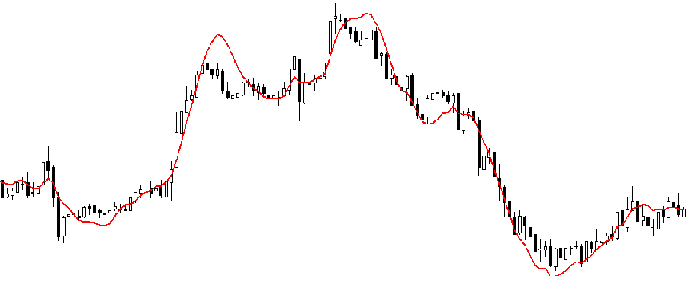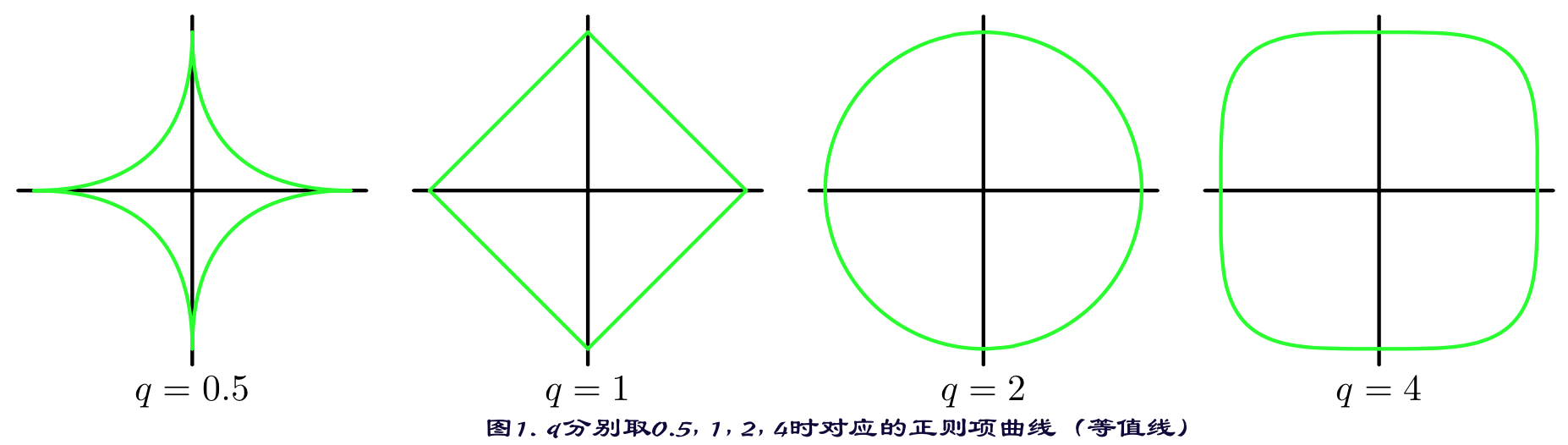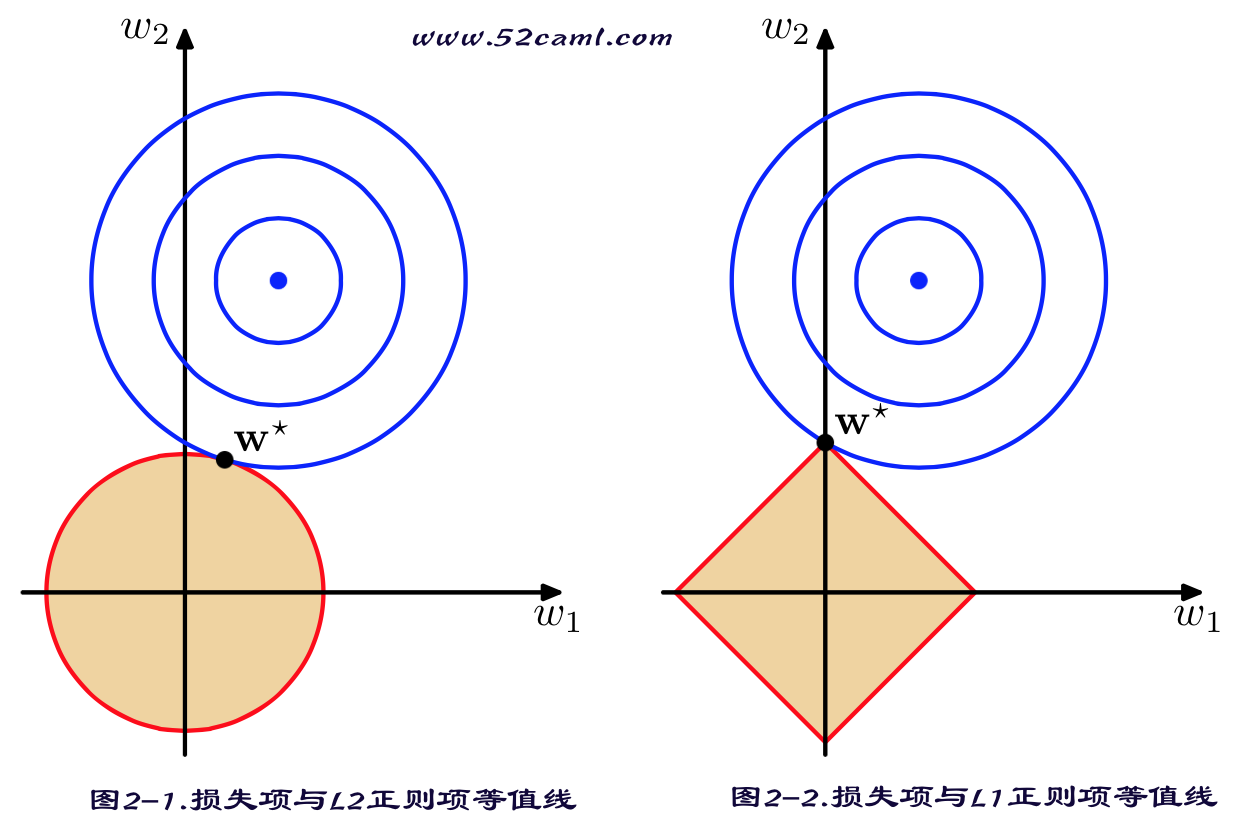• author: zhouyongsdzh@foxmail.com
• date: 2015-07-25
• weibo: @周永_52ML

### 符号定义

$x_j$ 第$j$维特征
$x$ 一条样本中的特征向量，$x=(1, x_1, x_2, \cdots, x_n)$
$x^{(i)}$ 第$i$条样本
$x_{j}^{(i)}$ 第$i$条样本的第$j$维特征
$y^{(i)}$ 第$i$条样本的结果（label）
$X$ 所有样本的特征全集，即$X=(x^{(1)},x^{(2)}, \cdots, x^{(m)})^T$
$Y$ 所有样本的label全集，即$Y=(y^{(1)},y^{(2)}, \cdots, y^{(m)})^T$
$w$ 参数向量，即$w=(w_0, w_1, \cdots, w_n)$
$w_j$ 第$j维$参数

# 写在前面

### 回归分析介绍

• 什么是回归分析？

回归分析是解决预测建模任务时的一种方法，用于研究自变量与因变量之间的关系。该方法主要用于预测、时间序列建模以及寻找变量之间的因果关系。 举例，rash driving和number of road accidents by a driver通过回归技术可以进行更好的研究。

回归分析是用于建模和数据分析的一个重要工具。这里，我们用曲线/直线去拟合数据点，希望所有数据点到曲线或直线的距离差异之和最小（后面会给出公式量化）。上图是某一阶段的股票指数波动示意图，用一条（红色）曲线拟合真实数据。

• 为什么要使用回归分析？

正如上面描述，回归分析多用于建立两个或多个变量之间的关系表达。我们通过一个例子理解这个问题：

假如，你想根据当前的经济环境预估企业的营收增长情况。公司最近的财报表明营收增长大约是经济增长的2.5倍。利用这个关系，就可以根据当前和过去的营收和经济数据，预测公司未来的营收增长情况。

使用回归分析有诸多好处，比如：

1. 它可以清晰的表示自变量（特征）与因变量（结果）之间的显著关系；
2. 还可以表明多个自变量（特征）对因变量（结果）的影响程度（根据feature对应权重大小）.

同时，回归分析也可以去比较两个变量之间的影响，比如促销活动的次数与价格波动的影响。这些有助于帮助市场研究人员/数据分析师/数据科学家去消除或评估最佳的一组变量用于建立预测模型。

• 回归技术分类

有很多种不同的回归技术可做预测。根据目标变量的个数、因变量的类型以及回归函数的形状这三个维度对回归技术做一个归类。我们从回归家族中拿出两个经典的回归技术——线性回归和逻辑斯蒂回归，详细阐述其技术原理和应用场景。

一睹为快，简要的看下二者在变量上的差异：

Logistic回归 连续或离散 (0,1)之间连续值 非线性

### 线性回归（Linear Regression）

#### 线性回归模型

• 模型表达

$$y(x, w) = w_0 + w_1 x_1 + \cdots + w_n x_n \qquad (ml.1.1.1)$$

其中，$x_1,x_2,\cdots,x_n$表示自变量（集合）；$y$是因变量；$w$为参数向量；$w_i$表示对应自变量（特征）的权重，$w_0$是偏倚项（又称为截距）。

关于参数$w$：

1. 在物理上可以这样解释：在自变量（特征）之间相互独立的前提下，$w_i$反映自变量$x_i$对因变量影响程度，$w_i$越大，说明$x_i$对结果$y$的影响越大。
2. 通过每个字变量（特征）前面的参数，可以很直观的看出哪些特征分量对结果的影响比较大。
3. 在统计中，$w_1,w_2,\cdots,w_n$称为偏回归系数，$w_0$称为截距。

如果令$x_0=1, y(x,w)=h_{w}(x)$, 可以将公式$(ml.1.1.1)$写成向量形式，即：

$$h_{w}(x) = \sum_{i=0}^{n} w_i x_i = w^T x \qquad(ml.1.1.2)$$

其中，$w=(w_0, w_1, \cdots, w_n)$，$x=(1, x_1, x_2, \cdots, x_n)$ 均为向量，$w^T$为$w$的转置。

公式$(ml.1.1.2)$中，假设特征空间与输入空间$x$相同。

准确的讲，模型表达式要建立的是特征空间与结果之间的关系。在一些应用场景中，需要将输入空间映射到特征空间，然后建模. 定义映射函数为$\phi(x)$，因此我们可以把公式$(ml.1.1.2)$写成更通用的表达方式：

$$h_w(x) = w^T \phi(x)$$

特征映射相关技术，包括特征哈希、特征学习、Kernel等，在后面的章节中会详细介绍。

• 参数学习准则

公式$(ml.1.1.2)$中的参数向量$w$是$n+1$维，每个参数的取值是实数集合，也就是说参数向量$w$在$n+1$维实数空间中取值结果有无穷种可能。

那么，如何利用一个规则或机制帮助我们评估求得的参数$w$，并且使得到的线性模型效果最佳？直观地认为，如果求得的参数\$w$线性求和后，得到的结果$h_{w}(x)$与真实值$y$之差越小越好。

这是我们需要引入一个函数用来衡量$h_{w}(x)$表示真实值$y$好坏的程度，该函数称为损失函数（loss function，也称为错误函数）。数学表示如下：

\begin{align} & J(w) = \frac{1}{2} \sum_{i=1}^{m} \left(h_{w}(x^{(i)}) - y^{(i)} \right)^2 \\\ & \min_{w} \quad J(w) \end{align} \qquad (ml.1.1.3)

这个损失函数用的是$x^{(i)}$的估计值$h_{w}(x^{(i)})$与真实值$y^{(i)}$之差的平方和。从优化的角度讲，公式$(ml.1.1.3)$是待优化的目标函数（Object Function）（如果不考虑其它问题，诸如过拟合等），可将其转化为最优化问题求参数。

#### 参数学习－线性回归目标函数

• 最小二乘法（Least Square）

最小二乘法是一种完全数学描述的方法，直接给出闭式解结果。它用$X$表示观测数据中的特征矩阵，结果表示成$Y$向量，目标函数仍是$(ml.1.1.3)$，那么$w$可直接用下面公式表示：

$$w = (X^T X)^{-1} X^T Y \qquad \qquad (ml.1.1.4)$$

公式来源：

$\qquad X^T X w = X^T Y$

• 梯度下降法（Gradient Descent）

由于最小二乘法直接进行矩阵运算（求逆等），尽管可以得到全局最优解。但是在互联网海量数据背景下的回归分析或预测问题，其计算效率较低，甚至无法完成（涉及超大矩阵的求逆运算）。

而基于梯度法求解参数是一个不错的选择，原因主要有2点：

1. 算法复杂度与样本规模（样本数$m$、特征维度$n$）呈线性关系；
2. 如果目标函数是凸函数，批梯度法可保证能得到最优解，随机梯度法也能近似得到最优解。

基于梯度法求解回归问题的目标函数极值问题，将在《最优化算法》系列中详细讲解。

• 最小二乘法与梯度下降法求解异同

• 相同点

1. 本质相同：两种求解方法都是在给定已知数据（自变量$x$，因变量$y$）的前提下对因变量$y$算出一个估值函数（$x与y$关联表达式），然后对给定的新输入$x$通过估值函数得出$y$。
2. 目标相同：都是在已知数据的框架下，使得估算值与真实值的之差的平方和尽可能小。
• 不同点

实现方法与结果不同：最小二乘法直接通过建立等价关系找到全局最小，非迭代法。而梯度下降法作为迭代法的一种，先给定一个参数向量初始值，然后向目标函数下降最快的方向调整（即梯度方向），在若干次迭代之后找到全局最小。

相比最小二乘法，随机梯度下降法的一个缺点是：在接近极值时收敛速度变慢，并且该方法对初始值的选取比较敏感。

#### 概率解释－回归模型目标函数

• 中心极限定理

目标函数的概率解释需要用到中心极限定理。中心极限定理本身就是研究独立随机变量和的极限分布为正态分布的问题。

中心极限定理公式表示：

设$n$个随机变量$X_1, X_2, \cdots, X_n$相互独立，均具有相同的数学期望与方差，即$E(X_i) = \mu$; $D(X_i) = \sigma^2$。令$Y_n$为随机变量之和，有

$$Y_n = X_1 + X_2 + \cdots + X_n \qquad (n.ml.1.1.1)$$

$$Z_n = \frac {Y_n - E(Y_n)} {\sqrt{D(Y_n)}} = \frac {Y_n - n \mu} {\sqrt{n} \sigma} \rightarrow \mathcal{N}(0,1) \qquad(n.ml.1.1.2)$$

称随机变量$Z_n$为$n$个随机变量$X_1, X_2, \cdots, X_m$的规范和。

中心极限定理定义：

设从均值为$\mu$、方差为$\sigma^2$（有限）的任意一个总体中抽取样本量为$n$的样本，当$n$充分大时，样本均值的抽样分布[$\frac{1}{n} Y_n$]近似服从于均值为$\mu$、方差为$\sigma^2$的正态分布。

• 高斯分布

假设给定一个输入样例$x^{(i)}$根据公式$(ml.1.1.1)$得到预测值$w^{T}x^{(i)}$与真实值$y^{(i)}$之间存在误差，即为$\epsilon^{(i)}$。那么，它们之间的关系表示如下：

$$y^{(i)} = w^T x^{(i)} + \epsilon^{(i)} \qquad (ml.1.1.5)$$

而这里假设误差$\epsilon^{(i)}$服从标准高斯分布是合理的。解释如下：

回归模型的最终目标是建立自变量$x$与结果$y$之间的关系（通过函数表达式），希望通过$x$能较准确的表示结果$y$。

而在实际应用场景中，很难甚至不可能把导致$y$结果的所有变量（特征）都找出来，并放到回归模型中。那么模型中存在的$x$通常认为是影响结果$y$最主要的变量集合（又称因子, 在ML中叫做特征集）。根据中心极限定理，把那些对结果影响比较小的变量（假设独立同分布）之和认为服从正态分布是合理的。

示例说明误差服从高斯分布是合理的：

Andrew Ng《机器学习》课程第1节的线性回归例子中，根据训练数据建立房屋的面积$x$与房屋的售价$y$之间的函数表达。

它的数据集中把房屋面积最为主要的变量。除此之外我们还知道房屋所在的地段（地铁、学区、城区、郊区），周边交通状况，当地房价，楼层，采光，绿化面积，… 等等诸多因素会影响房价。

在实际中，因数据收集问题可能拿不到所有影响房屋售价的因素（变量），可以假设多个因素变量相互独立，根据中心极限定理，认为变量之和服从高斯分布。即：

$$\epsilon^{(i)} = y^{(i)} - w^T x^{(i)} \rightarrow \mathcal{N}(0,\sigma^2) \qquad(n.ml.1.1.3)$$

那么$x$和$y$的条件概率可表示为：

$$p(y^{(i)} | x^{(i)}; w) = \frac{1}{\sqrt{2\pi} \sigma} \exp {\left(- \frac{(y^{(i)} - w^T x^{(i)})^2}{2 \sigma^2}\right)} \qquad(ml.1.1.6)$$

• 极大似然估计与损失函数极小化等价

根据公式$(ml.1.1.6)$估计得到一条样本的结果概率，模型的最终目标是希望在全部样本上预测最准，也就是概率积最大，这个概率积就是似然函数。优化的目标的函数即为似然函数，表示如下：

$$\max_{w} \quad L(w) = \prod_{i=1}^{m} \frac{1}{\sqrt{2\pi} \sigma} \exp \left(- \frac{(y^{(i)} - w^T x^{(i)})^2} {2 \sigma^2}\right) \qquad(ml.1.1.7)$$

对$L(x)$取对数，可得：

$$\max_{w} \; \mathcal{l}(w) = -m \cdot \log \sqrt{2\pi} \sigma - \frac{1}{2\sigma^2} \sum_{i=1}^{m} \left(y^{(i)} - w^T x^{(i)}\right)^2 \qquad(ml.1.1.8)$$

由于$n,\sigma$变量为常数，因此公式$(ml.1.1.8)$等价于：

$$\min_{w} \; \frac{1}{2} \sum_{i=1}^{m} \left(y^{(i)} - w^T x^{(i)}\right)^2 \qquad(ml.1.1.9)$$

我们可以发现，经过最大似然估计推导出来的待优化的目标函数$(ml.1.1.9)$与平方损失函数$(ml.1.1.3)$是等价的。因此，可以得出结论：

线性回归 误差平方损失极小化与极大似然估计等价。其实在概率模型中，目标函数的原函数（或对偶函数）极小化（或极大化）与极大似然估计等价，这是一个带有普遍性的结论。

在本系列《第02章：深入浅出ML之Entropy-Based家族》和李航老师的《统计学习方法》中，谈到最大熵模型时，都给出了对偶函数极大化与极大似然估计等价的结论。

为什么是条件概率？

因为我们希望预测值与真实值更接近，这就意味着希望求出来的参数$w$，在给定输入$x$的情况下，得到的预测值等于真实值的可能性越大越好。而$w, x$均是前提条件，因此用条件概率$p(y|x; w)$表示。即$p(y|x; w)$越大，越能说明估计的越准确。（当然也不能一味地只优化该条件概率，还要考虑拟合过度以及模型的泛化能力问题，这部分在《第07章：深入浅出ML之统计学习理论》中详细阐述。）

### 回归模型与正则化

$$\min_{w} \quad J(w) = \sum_{i=1}^{m} \left(h_{w}(x^{(i)}) - y^{(i)} \right)^2 \qquad (n.ml.1.1.4)$$

$$\min_{w} \quad \sum_{i=1}^{m} \left(w^T x^{(i)} - y^{(i)} \right)^2 + {\lambda} {\Vert w \Vert}_{2}^2 \qquad (ml.1.1.10)$$

Ridge Regression，又称Tikhonov Regularization，由1943年苏联人Tikhonov发表。$$\min_{w} \quad \sum_{i=1}^{m} \left(w^T x^{(i)} - y^{(i)} \right)^2 + \lambda {\Vert w \Vert}_{1} \qquad (ml.1.1.11)$$

L1与L2

L1范数与L2范数正则化都有助于降低过拟合风险，但L1还会带来一个额外的好处，就是L1正则化更易于获得“稀疏”（sparse）解，即它求得的参数w会有更少的非零分量。### 逻辑斯蒂回归（Logistic Regression）

#### 逻辑斯蒂分布

• 逻辑斯蒂分布

设$X$是连续随机变量，如果随机变量$X$对应的概率密度函数$f(x)$和累积分布函数$F(x)$分别是：

$$f(x) = \frac{e^{- \frac{x-\mu}{s}}} {s(1+e^{- \frac{x-\mu}{s}})^2} \qquad\quad(ml.1.1.10)$$

$$F(x) = P(X \leq x) = \int_{-\infty}^{x} f(x) dx = \frac {1} {1+e^{- \frac{x-\mu}{s}}} \qquad(ml.1.1.11)$$

那么，$X$服从逻辑斯蒂分布。其中，$\mu$为位置参数，$s>0$为形状参数。

累积分布函数属于逻辑斯蒂函数，其图形是一条$S$型曲线（又称为Sigmoid曲线）。该曲线的特点是：以点$(\mu, \frac{1}{2})$为中心对称，即满足：

$$F(-x+\mu) - \frac{1}{2} = -F(x-\mu) + \frac{1}{2} \qquad(n.ml.1.1.4)$$

曲线在中心附近增长较快，在两端增长速度较慢。从密度函数和分布函数图形都能看出，形状参数$s$的值越小，曲线在中心附近增长的越快。

#### 逻辑斯蒂回归模型

1. 回归模型是连续型模型，即预测出的值都是连续值（实数值），非离散值；
2. 预测结果受样本噪声的影响比较大。

• LR模型表达式

LR模型表达式为参数化的逻辑斯蒂（累积）分布函数（默认参数$(\mu=0,s=1$）即：

$$h_w(x) = \frac{1}{1+e^{-w^T \cdot x}} \qquad(ml.1.1.12)$$

$h_w(x)$作为事件结果$y=1$的概率取值。这里，$x \in R^{n+1}, y \in \{1,0\}$，$w \in R^{n+1}$是权值向量。其中权值向量$w$中包含偏置项，即$w=(w_0, w_1, \cdots, w_n)$, $x=(1, x_1, \cdots, x_n)$。

_问题：LR输出结果是否可以是概率值？_

• 理解LR模型

• 对数几率

一个事件发生的几率(odds)是指该事件发生的概率与该事件不发生的概率的比值。如果事件发生的概率是$p$，那么该事件的几率为$\frac{p}{1-p}$，该事件的对数几率（log odds，用logit函数表示）是：

$$logit(p) = \log \frac{p}{1-p} \qquad(ml.1.1.13)$$

对LR而言，根据公式$(ml.1.1.12)$和$(ml.1.1.13)$可得：

$$\log \frac{h_w(x)}{1-h_w(x)} = w^T x \qquad(ml.1.1.14)$$

即在LR模型中，输出$y=1$的对数几率是输入实例$x$的线性函数。

• 函数映射

除了从对数几率的角度理解LR外，从函数映射也可以理解LR模型：

考虑对输入实例$x$进行分类的线性表达式$w^Tx$，其值域为实数域（$x \in R^{n+1}$，$w \in R^{n+1}$）。通过LR模型表达式$(ml.1.1.13)$可以将线性函数$w^T x$的结果映射到$(0,1)$区间，取值表示为结果为1的概率（在二分类场景中）。

线性函数的值愈接近正无穷$\infty$，概率值就越接近1；反之，其值越接近负无穷，概率值就越接近0。这样的模型就是LR模型。

逻辑斯蒂回归本质上还是线性回归，只是特征到结果的映射过程中加了一层函数映射（即sigmoid函数），即先把特征/变量线性求和，然后使用sigmoid函数将线性和约束至$(0,1)$之间，结果值用于二分或回归预测。

• LR模型——概率解释

LR模型多用于解决二分类问题，如广告是否被点击（是/否）、商品是否被购买（是/否）等互联网领域中常见的应用场景。

但是实际场景中，我们又不把它处理成“绝对的”分类问题，而是用其预测值作为事件发生的概率。

这里从事件、变量以及结果的角度给予解释。

我们所能拿到的训练数据统称为观测样本。问题：样本是如何生成的？

一个样本可以理解为发生的一次事件，样本生成的过程即事件发生的过程。对于0/1分类问题来讲，产生的结果有两种可能，这符合伯努利试验的概率假设。因此，我们可以说样本的生成过程即为伯努利试验过程，产生的结果（0/1）服从伯努利分布。这里我们假设结果为1的概率为$h_{w}(x)$，结果为0的概率为$1-h_{w}(x)$

那么，对于第$i$个样本，概率公式表示如下：

$$P(y^{(i)}=1|x^{(i)}; w) = h_{w}(x^{(i)}) \qquad (ml.1.1.15)$$

$$P(y^{(i)}=0|x^{(i)}; w) = 1 - h_{w}(x^{(i)}) \qquad (ml.1.1.16)$$

将公式$(ml.1.1.15)$和$(ml.1.1.16)$合并在一起，可得第$i$个样本正确预测的概率：

$$P(y^{(i)}|x^{(i)}; w) = (h_{w}(x^{(i)}))^{y^{(i)}} \cdot (1 - h_{w}(x^{(i)}))^{1-y^{(i)}} \qquad (ml.1.1.17)$$

上式是对一条样本进行建模的数据表达。对于多条样本，假设每条样本生成过程独立，在整个样本空间中（$m$个样本）的概率分布为：

$$P(Y|X; w) = \prod_{i=1}^{m} \left( (h_{w}(x^{(i)}))^{y^{(i)}} \cdot (1 - h_{w}(x^{(i)}))^{1-y^{(i)}} \right) \qquad(ml.1.1.18)$$

通过极大似然估计（Maximum Likelihood Evaluation，简称MLE）方法求概率参数。具体地，下面给出了通过随机梯度下降法（Stochastic Gradient Descent，简称SGD）求参数。

_问题：公式$(ml.1.1.17)$是怎么得到的？_

可以从概率建模的角度给出解释

sigmoid函数在深度学习模型中常用作激活函数。

• 参数学习算法

公式$(ml.1.1.18)$不仅可以理解为在已观测的样本空间中的概率分布表达式。如果从统计学的角度可以理解为参数$w$似然性的函数表达式（即似然函数表达式）。参数在整个样本空间中的似然函数可表示为：

\begin{align} L(w) & = P(Y|X; w) \\\ & = \prod_{i=1}^{m} P(y^{(i)}|x^{(i)}; w) \\\ & = \prod_{i=1}^{m} \left( (h_{w}(x^{(i)}))^{y^{(i)}} \cdot (1 - h_{w}(x^{(i)}))^{1-y^{(i)}} \right) \end{align} \quad\qquad (ml.1.1.19)

为了方便参数求解，对公式$(ml.1.1.19)$取对数，可得：

\begin{align} l(w) & = logL(w) \\\ & = \sum_{i=1}^{m} \left( y^{(i)} \cdot \log (h_{w}(x^{(i)})) + (1-y^{(i)}) \cdot \log(1- h_{w}(x^{(i)})) \right) \end{align} \qquad (ml.1.1.20)

最大化log似然函数，就是最小化交叉熵误差（Cross Entropy Error）。

先不考虑累加和$\sum_{i=1}^{m}$，针对每个参数$w_j$求偏导：

\begin{align} \frac{\partial}{\partial w_j} l(w) & = \left( y \frac{1}{h_{w}(x)} - (1-y) \frac{1}{1-h_{w}(x)}\right) \frac{\partial}{\partial w_j} h_{w}(x) \\\ & = \left( \frac{y-h_{w}(x)}{h_{w}(x) \cdot (1 - h_{w}(x))}\right) \cdot h_{w}(x) (1 - h_{w}(x)) \cdot \frac{\partial}{\partial w_j} w^T x \\\ & = \left( y-h_{w}(x) \right) \cdot \frac{\partial}{\partial w_j} w^T x \\\ & = \left( y-h_{w}(x) \right) \cdot x_{j} \end{align} \qquad (ml.1.1.21)

最后，通过扫描样本，迭代下述公式可求得参数：

$$w_{j+1} = w_j + \alpha \cdot (y^{(i)} - h_{w}(x^{(i)})) \cdot x_{j}^{(i)} \qquad (ml.1.1.22)$$

公式$(ml.1.1.22)$中的$\alpha$表示学习率（learning rete，又称学习步长）。

除此之外，还有Batch GD，共轭梯度，拟牛顿法（LBFGS），ADMM分布学习算法等都可用于求解参数，这些将在《最优化算法》系列中的对应章节中详细介绍。

基于梯度法求目标函数极值，另一种推导方式：

\begin{align} l(w) & = \log L(w) \\\ & = \sum_{i=1}^{m} \left( y^{(i)} \cdot \log (h_{w}(x^{(i)})) + (1-y^{(i)}) \cdot \log(1- h_{w}(x^{(i)})) \right) \\\ & = \sum_{i=1}^{m} \left( y^{(i)} \cdot \underline { \log {\frac {h_w(x^{(i)})}{ 1-h_w(x^{(i)})}} } + \log (1-h_w(x^{(i)}))\right) \\\ & = \sum_{i=1}^{m} \left(y^{(i)} \cdot w^T x^{(i)} - \log (1 + \exp(w^Tx^{(i)})) \right) \end{align} \qquad(n.ml.1.1.5)

同样的，对每个参数求偏导，推导结果为：

$$\frac{\partial}{\partial w_j} l(w) = y^{(i)} x_j^{(i)} - \frac {\exp({w^Tx^{(i)}})} {1+\exp({w^Tx^{(i)}})} x_j^{(i)} = \left( y^{(i)} - h_{w}(x^{(i)}) \right) \cdot x_{j}^{(i)} \qquad(n.ml.1.1.6)$$

Sigmoid函数性质：

①. $h_w(-x) = 1 - h_w(x)$

②. $h_w^{\prime}(x) = (1-h_w(x)) \cdot h_w(x)$

推导：

\begin{align} h^{\prime}(x) & = (\frac {1}{1+e^{-x}})^{\prime} = - \frac {1}{(1+e^{-x})^2} \cdot (e^{-x})^{\prime} \\\ & = \frac {e^{-x}} {(1+e^{-x})^2} = \frac {e^{-x}} {1+e^{-x}} \cdot \frac{1} {1+e^{-x}} \\\ & = (1-h(x)) \cdot h(x) \end{align}

同时也可以得到$\frac{\partial \log h_w(x)}{\partial x} = (1-h_w(x))$，$\frac{\partial \log (1-h_w(x))}{\partial x} = -h_w(x)$

重要考察点

注：这部分公式推导是LR模型的核心部分，在机器学习相关面试中，LR模型公式推导是可能是考察频次最高的一个点。打算寻求数据挖掘、机器学习等职位的朋友，建议能做到公式的熟练推导。

• 交叉熵误差

其实从公式$(ml.1.1.20)$中不难看出，LR模型的对数似然函数对应的就是交叉熵的表达式。在给定样本空间下，对于条件概率$P(y|x)=h_w(yx)$来说，其似然函数表达式为：

$$\max_{h} likelihood(h) \propto \prod_{i=1}^{m} h_w(y^{(i)} x^{(i)}) \qquad (ml.1.1.23)$$

上式表示函数$h$的可能性，即$likelihood(h)$。该式越大，说明$h$越逼近真实目标函数$f$。将其转化为求极小值问题（添加负号），并写成$\log$形式为：

\begin{align} & \min_w \quad \frac{1}{m} \sum_{i=1}^{m} - \log (h_w(y^{(i)} x^{(i)})) \qquad\qquad(1) \\\ \Longrightarrow \; & \min_w \quad \frac{1}{m} \sum_{i=1}^{m} \underline{ \log (1+\exp(-y^{(i)} w^T x^{(i)})) } \quad\;\,(2)\\\ \Longrightarrow \; & \min_w \quad \underbrace { \frac{1}{m} \sum_{i=1}^{m} \underline{err(w,y^{(i)},x^{(i)})} }_{E_{in}(w)} \qquad\qquad\quad\, (3) \\\ \end{align} \qquad (ml.1.1.24)

公式$(1)$添加了$\frac{1}{m}$是为了写成Loss Function的长相（对于给定数据来说，其作为一个常数，对求解无妨）；公式$(3)$表达式又称为交叉熵损失（Cross-Entropy Error）。

值得说明的是：不止LR模型的损失函数是交叉熵损失，几乎所有的条件概率模型对应的Loss Function都是交叉熵损失。

#### LR模型与广义线性模型、最大熵模型、指数族分布

• LR模型是广义线性模型的特例

当目标值分布服从伯努利分布时

• LR模型是最大熵模型的特例

最大熵模型是基于最大熵原理（见《第2章：深入浅出ML之Entropy Methods家族》），优化条件概率$p(y|x)$熵，并通过对偶函数极大化或极大似然估计得到的概率模型。当$y$满足二项分布时，得到的概率模型即为$P(y=1|x)$.

• LR模型满足指数族分布

LR模型与指数族分布也存在密切的关系

指数族分布的归一化形式（Canonical Form）：

$$p(y|\eta) = h(y) \cdot g(\eta) \cdot \exp \{ \eta^T \mu(y) \} \qquad(n.ml.1.1.7)$$

前面说道，LR模型对应的结果只有两种可能，多次独立同分布实验服从二项分布。LR模型是指数族分布中$y$服从二项分布的特例。

#### LR模型在工业界的应用

• 模型易用：LR模型建模思路清晰，容易理解与掌握；
• 概率结果：输出结果可以用概率解释（二项分布），天然的可用于结果预估问题上；
• 强解释性：特征（向量）和标签之间通过线性累加与Sigmoid函数建立关联，参数的取值直接反应特征的强弱，具有强解释性；
• 简单易用：有大量的机器学习开源工具包含LR模型，如sklearn、spark-mllib等，使用起来比较方便，能快速的搭建起一个learning task pipeline；

1. 学习的过拟合问题
2. 学习的数据稀疏性问题
3. 模型自身的学习效率（收敛速度，稳定性）
4. 训练模型时数据、特征的扩展性问题，即学习算法可否在分布式环境下工作；
5. 如何结合实际应用场景（比如多资源位／多广告位的点击预估问题），给出相应的解决方案.

• loss function + $\underline{ \lambda |w|_{2}^{2}}$：解决过拟合

• loss function + $\underline{ \lambda |w|_{1}}$：解决稀疏性，比如Google13年出的预估方法－FTRL模型，虽然是在线学算法，但主要是为了解决预估时的稀疏性问题。

1. ADMM算法在拉格朗日函数中引入惩罚函数项（二阶项）用于保证求解时的收敛效率（收敛速度）和结果的健壮性（放松目标函数为强凸的限制）。
2. 目标函数可分的，可以将数据集划分多了数据block，各自学习得到局部参数，然后汇总得到全局参数；进一步将全局参数“广播”（broadcast）至各个计算节点，用于下一轮局部参数学习的初始值。
3. ADMM算法框架将目标函数划分为两部分（为了引入全局参数），局部参数与全局参数的组合作为约束条件；算法自身结构也是为了适应在分布式环境下求解。

1. 特征离散化表示后（尤其是ID类特征），特征会非常稀疏，学习时需要考虑稀疏性问题；
2. 训练数据集相比样本的高维度特征向量表示来说，显得“捉襟见肘”时，学习时要考虑过拟合问题；
3. 如何在更多的训练数据集和更高的数据特征维度上，借助分布式框架＋优化求解算法框架解决超大规模离散LR模型学习问题？

### 回归问题相关解释

• 广义线性回归

其实，回归家族的模型可以统称为广义上的线性回归。如果把$w$看作是参数，而$x_i, x_i x_j, x^2_i$等看作参数的常量（他们可直接从线性观测数据中计算得到），如此上面介绍的回归模型都可以看作是参数$w$的线性函数。

• 线性回归与线性关系

线性回归固然可以表达线性关系，但是也可以表达非线性关系。如<广义线性回归>中解释的那样，如果先把每个特征变量映射到一个函数（如$x_i \rightarrow x^2_i$），然后再进行线性计算。如此，线性回归可以表达特征与结果之间的非线性关系。

广义线性回归既可以表达线性关系，也可以表达非线性关系。

### Next …

• Ridge Regression
• Lasso Regression
• Softmax Regression
• 广义线性模型